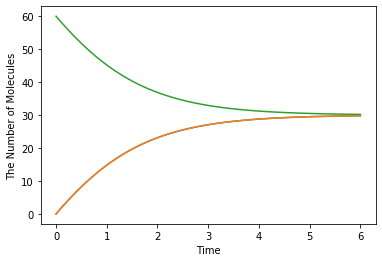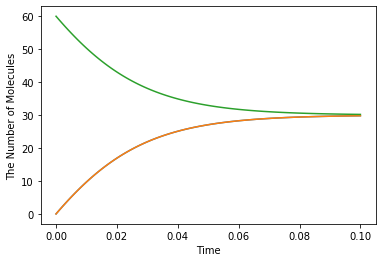# How to Use the Unit System¶

Important: The unit system is a feature under development. The following section might not work properly yet.

Here, we show some examples using the unit system in ecell4. This feature requires Python library, pint. Install pint before running this example as follows: pip install pint. See also the website https://pint.readthedocs.io/en/latest/.

In :
%matplotlib inline
from ecell4.prelude import *


## With no units¶

First, imagine a very simple system only with binding and unbinding reactions like:

In :
with species_attributes():
A | B | C | {'D': 1, 'radius': 0.005}

with reaction_rules():
A + B == C | (0.01, 0.3)

m = get_model()


The species_attributes section defines a diffusion constant and radius of Species, A, B and C. For example, the diffusion rate of A is 1, and its dimensionality is expected to be [length**2/time]. However, what is the scale? Is it meter? Or mile?

Once the base units are determined, e.g. micrometer as [length] and second as [time], all the units must be consistent within the model. The second order rate consant must have dimensionality [1/(substance/length**3)/time], which is micrometer**3/item/second. Thus, when the parameter is given as 1/molar/min in some literature, you have to translate it by yourself.

In :
show(m)

A | {'D': 1, 'radius': 0.005}
B | {'D': 1, 'radius': 0.005}
C | {'D': 1, 'radius': 0.005}

A + B > C | 0.01
C > A + B | 0.3


## Introducing units¶

ecell4 provides the way to handle units in the modeling environment. Here is an example.

In :
from ecell4.extra.unit import getUnitRegistry
ureg = getUnitRegistry()
Q_ = ureg.Quantity


First, create your own unit system,ureg, by using ecell4.extra.unit.getUnitRegistry. With this UnitRegistry, you can make a quantity with its unit as ureg.Quantity(value, unit). (Please be careful about the type of Quantity. It looks same with Quantity given by pint, but is slightly changed in ecell4 though all the original functionality in pint is availble even in ecell4. Please not use ureg = pint.UnitRegistry().)

In :
with species_attributes():
A | B | C | {'D': Q_(1, 'um**2/s'), 'radius': Q_(0.005, 'um')}

with reaction_rules():
A + B == C | (Q_(0.01, '1/(item/um**3)/s'), Q_(0.3, '1/s'))

m = get_model()


The default base units are meter for [length], second for [time], and item (which means the number of molecules) for [substance]. When you change the default base unit, do like ureg = getUnitRegistry(length='micrometer').

In :
show(m)

A | {'D': Quantity_Real(1e-12, 'meter ** 2 / second'), 'radius': Quantity_Real(5e-09, 'meter')}
B | {'D': Quantity_Real(1e-12, 'meter ** 2 / second'), 'radius': Quantity_Real(5e-09, 'meter')}
C | {'D': Quantity_Real(1e-12, 'meter ** 2 / second'), 'radius': Quantity_Real(5e-09, 'meter')}

A + B > C | Quantity_Real(1e-20, 'meter ** 3 / item / second')
C > A + B | Quantity_Real(0.3, '1 / second')


Now you can provide quantities in any unit regardless of the base units.

In :
with species_attributes():
A | B | C | {'D': Q_(1e-8, 'cm**2/s'), 'radius': Q_(5, 'nm')}

with reaction_rules():
A + B == C | (Q_(6.02214129, '1/uM/s'), Q_(18, '1/min'))

m = get_model()
show(m)

A | {'D': Quantity_Real(1e-12, 'meter ** 2 / second'), 'radius': Quantity_Real(5e-09, 'meter')}
B | {'D': Quantity_Real(1e-12, 'meter ** 2 / second'), 'radius': Quantity_Real(5e-09, 'meter')}
C | {'D': Quantity_Real(1e-12, 'meter ** 2 / second'), 'radius': Quantity_Real(5e-09, 'meter')}

A + B > C | Quantity_Real(1e-20, 'meter ** 3 / item / second')
C > A + B | Quantity_Real(0.3, '1 / second')


You can operate quantities, and make a new quantity. See https://pint.readthedocs.io/en/latest/ for more details.

In :
volume = Q_(1, 'fL')
conc = Q_(100, 'nM')
print((volume * conc).to('item'))

60.221412900000004 item


In addition to the model creation, run_simulation (and ensemble_simulations) also supports the unit system.

In :
run_simulation(Q_(0.1, 'min'), y0={'C': Q_(60, 'item')}, volume=Q_(1, 'fL'), model=m, solver='ode')Even if you change the base units, the behavior of simulations is kept consistent. In the following example, base units are rescaled to micrometer and minute with no change in the modeling section.

In :
ureg = getUnitRegistry(length='micrometer', time='minute')
Q_ = ureg.Quantity

with species_attributes():
A | B | C | {'D': Q_(1e-8, 'cm**2/s'), 'radius': Q_(5, 'nm')}

with reaction_rules():
A + B == C | (Q_(6.02214129, '1/uM/s'), Q_(18, '1/min'))

m = get_model()
show(m)
run_simulation(Q_(0.1, 'min'), y0={'C': Q_(60, 'item')}, volume=Q_(1, 'fL'), model=m, solver='ode')

A | {'D': Quantity_Real(60, 'micrometer ** 2 / minute'), 'radius': Quantity_Real(0.005, 'micrometer')}
B | {'D': Quantity_Real(60, 'micrometer ** 2 / minute'), 'radius': Quantity_Real(0.005, 'micrometer')}
C | {'D': Quantity_Real(60, 'micrometer ** 2 / minute'), 'radius': Quantity_Real(0.005, 'micrometer')}

A + B > C | Quantity_Real(0.6, 'micrometer ** 3 / item / minute')
C > A + B | Quantity_Real(18, '1 / minute')## Checking dimensionality¶

This feature is still being developed. Please report issues when getting the wrong behavior.

You can check units in the model by ecell4.extra.unit.check_model.

For example, the dimensionality of a diffusion constant must be [length**2/time]. When you give a unit with a wrong dimensionality, an exception DimensionalityMismatchError would be thrown as:

In :
from ecell4.extra.unit import check_model, DimensionalityMismatchError
ureg = getUnitRegistry()
Q_ = ureg.Quantity

In :
with species_attributes():
A | {'radius': Q_(0.005, 'um'), 'D': Q_(1.0, 'um/s')}

try:
check_model(get_model())
except DimensionalityMismatchError as e:
print('{}: {}'.format(e.__class__.__name__, e))

DimensionalityMismatchError: Attribute [D] in Species [A] has wrong dimensionality. [[length]**2/[time]] is expected.


When checking dimensionality of units in the model by check_model, you can omit no unit.

In :
with species_attributes():
A | {'radius': 0.005, 'D': Q_(1.0, 'um**2/s')}

try:
check_model(get_model())
except DimensionalityMismatchError as e:
print('{}: {}'.format(e.__class__.__name__, e))

DimensionalityMismatchError: Attribute [radius] in Species [A] has wrong dimensionality. [[length]] is expected.


A kinetic rate constant of reactions is verified based on the order of the reaction. The first order reaction rate should have [1/time], and the second order should have [l/(substance/length**3)/time] in volume.

In :
with reaction_rules():
A + B > C | Q_(0.3, '1/s')

try:
check_model(get_model())
except DimensionalityMismatchError as e:
print('{}: {}'.format(e.__class__.__name__, e))

DimensionalityMismatchError: ReactionRule [A+B>C|0.3] has wrong dimensionality [1 / [time]]. [[length]**3/[substance]/[time]] is expected.


The dimensionality of a synthetic reaction depends on the dimension which the products belongs to.

In :
with reaction_rules():
~A > A | Q_(0.3, '1/s')

try:
check_model(get_model())
except DimensionalityMismatchError as e:
print('{}: {}'.format(e.__class__.__name__, e))

DimensionalityMismatchError: ReactionRule [>A|0.3] has wrong dimensionality [1 / [time]]. [[substance]/[time]/[length]**3] is expected.


An unit of the reaction rate between a molecule and structure is also tested.

In :
with species_attributes():
B | {'location': 'M'}
M | {'dimension': 2}

with reaction_rules():
A + M > B | Q_(0.3, '1/s')

try:
check_model(get_model())
except DimensionalityMismatchError as e:
print('{}: {}'.format(e.__class__.__name__, e))

DimensionalityMismatchError: ReactionRule [A+M>B|0.3] has wrong dimensionality [1 / [time]]. [[length]**1/[time]] is expected.


Additionally, rate law representations accept quantities with a unit too. See the example below:

In :
with reaction_rules():
S > P | Q_(1.0, 'uM/s') * S / (Q_(100, 'nM') + S)

check_model(get_model())


Here, the reaction above has two quantities, Vmax = Q_(1.0, 'uM/s') and Km = Q_(100, 'nM'). First, Km must have the same dimensionality with S, which is [concentration].

In :
with reaction_rules():
S > P | Q_(1.0, 'uM/s') * S / (Q_(100, 'nM/s') + S)

try:
check_model(get_model())
except DimensionalityMismatchError as e:
print('{}: {}'.format(e.__class__.__name__, e))

DimensionalityMismatchError: Failed to evaluate [((Quantity_Real(6.02214129e+20, 'item / meter ** 3 / second') * S) / (Quantity_Real(6.02214129e+19, 'item / meter ** 3 / second') + S))] (1*S>1*P|0). Cannot convert from 'item / meter ** 3 / second' ([substance] / [length] ** 3 / [time]) to 'item / meter ** 3' ([substance] / [length] ** 3)


Secondly, the dimensionality of a rate equation must be [concentration/time]. Therefore, the dimensionality of Vmax should be [concentration/time] too.

In :
with reaction_rules():
S > P | Q_(1.0, '1/s') * S / (Q_(100, 'nM') + S)

try:
check_model(get_model())
except DimensionalityMismatchError as e:
print('{}: {}'.format(e.__class__.__name__, e))

DimensionalityMismatchError: ReactionRule [1*S>1*P|0] has wrong dimensionality [1 / [time]]. [[substance]/[length]**3/[time]] is expected.


When you give a value with no unit, it is regarded as dimensionless.

In :
with reaction_rules():
S > P | 10.0 * Q_(0.1, 'uM/s') * S**2 / (Q_(100, 'nM')**2 + S**2)

m = get_model()
show(m)
check_model(m)

S > P | ((Quantity_Real(6.02214129e+20, 'item / meter ** 3 / second') * pow(S, 2)) / (Quantity_Real(3.626618571672287e+39, 'item ** 2 / meter ** 6') + pow(S, 2)))